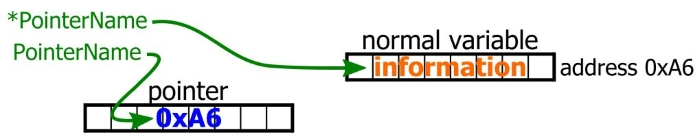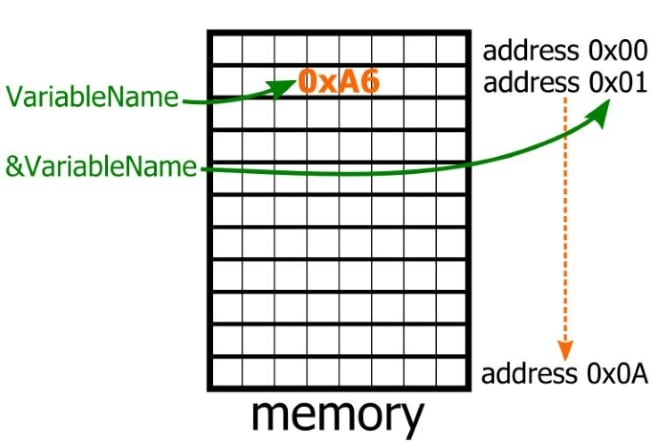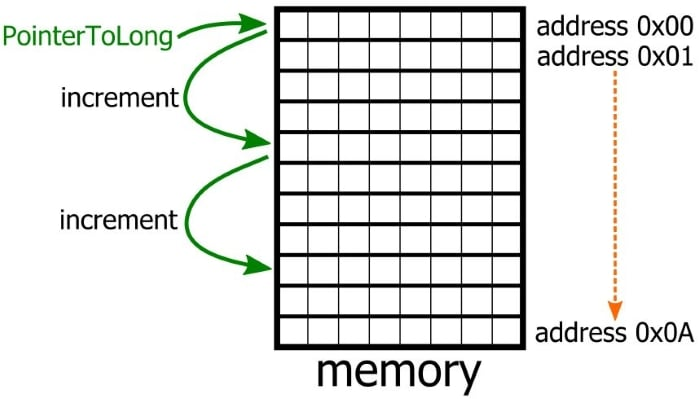Technical Article

# How to Use Pointers in C-Language Firmware

January 25, 2019 by Robert Keim

## In this article we’ll discuss pointer operators, pointer arithmetic, and two situations in which pointers can improve your code.

In this article we’ll discuss pointer operators, pointer arithmetic, and two situations in which pointers can improve your code.

### Working with Pointer Values

#### Modifying and Dereferencing Pointers

There are two values associated with a pointer. The first is the memory address that is stored in the pointer itself, and the second is the data that is stored at this memory address. To modify the address stored in the pointer variable, you simply use the equals sign:

RxByte_ptr = 0x40;

To access the data stored at the pointer’s address, you use an asterisk. This works for both reading and writing.

ReceivedData = *RxByte_ptr;
*TxByte_ptr = TransmitData;

Accessing the value at which a pointer points is called dereferencing, and the asterisk (when used with pointers) is called the dereference operator.#### Obtaining the Address of a Variable

An important detail related to the use of pointers is the C “address-of” operator; the symbol for this is &. Though the & is attached to normal variables rather than pointers, I still consider it a “pointer operator” because its use is so closely related to pointer implementation.

When an & is placed in front of a variable name, the program uses the address of the variable rather than the value of the variable.This enables you to place the address of a variable in a pointer even though you have no idea where in memory a particular variable will be located. The use of the & operator is demonstrated in the following code snippet, which also serves as a summary of basic pointer usage.

char DisplayChar;
char TestingVariable;
char *DisplayChar_ptr;

DisplayChar = 0x41;
DisplayChar_ptr = &DisplayChar;
TestingVariable = *DisplayChar_ptr;
*DisplayChar_ptr = 0x42;
TestingVariable = DisplayChar;

Here’s a step-by-step description of what this code is doing:

DisplayChar = 0x41;

The DisplayChar variable now holds the value corresponding to ASCII ‘A’.

DisplayChar_ptr = &DisplayChar;

The pointer (DisplayChar_ptr) now holds the address of the variable DisplayChar. We have no idea what this address is—i.e., we do not know the number that is stored in DisplayChar_ptr. Furthermore, we don’t need to know; this is the compiler’s business, not ours.

TestingVariable = *DisplayChar_ptr;

TestingVariable now holds the value of the DisplayChar variable, namely, 0x41.

*DisplayChar_ptr = 0x42;

We have just used the pointer to modify the value stored in the address corresponding to the DisplayChar variable; it now has 0x42, which is ASCII ‘B’.

TestingVariable = DisplayChar;

TestingVariable now holds the value 0x42.

### Pointer Arithmetic

Most of the time, a C variable holds a value that can vary, and pointer variables are no exception. Common arithmetic operations that are used to modify the value of a pointer are addition (e.g., TxByte_ptr = TxByte_ptr + 4), subtraction (TxByte_ptr = TxByte_ptr - 4), increment (TxByte_ptr++), and decrement (TxByte_ptr--). It is possible to subtract one pointer from another, as long as the two pointers have the same data type. However, you cannot add one pointer to another pointer.

Pointer arithmetic is not quite as straightforward as it seems. Let’s say that you have a pointer with the data type of long. You’re debugging some code and you’re currently single-stepping through a routine that repeatedly increments this pointer. You notice in your Watch window that the value of the pointer does not increase by one with every increment. What’s going on here?

If you can’t readily think of the answer, you should spend a bit more time pondering the nature of pointers. The pointer in this code is used with long variables, i.e., variables that consume four bytes of memory. When you increment the pointer, you don’t actually want the value of the pointer to increase by one memory location (we’re assuming here that the memory is organized as bytes). Rather, you want it to increase by four memory locations, so that it points to the next long variable. The compiler knows this, and it modifies the value of the pointer accordingly.The same thing happens when you add a number to a pointer or subtract a number from a pointer. The address stored in the pointer will not necessarily increase or decrease by that number; rather, it will increase or decrease by that number multiplied by the size in bytes of the pointer’s data type.

### Pointers and Arrays

Pointers and arrays are closely related. When you declare an array, you are essentially creating a constant pointer that always holds the array’s starting address, and the index notation that we use to access elements in an array can also be used with pointers.

For example, let’s say that you have a char pointer named TxBuffer that currently holds the address 0x30. The following code snippet shows two equivalent ways of accessing the data at address 0x31.

TxByte = *(TxBuffer + 1);
TxByte = TxBuffer;

### When to Use Pointers

In this section I want to briefly discuss two coding situations that can benefit from the use of pointers and that are particularly relevant to embedded applications.

#### Pointer vs. Array

The first follows naturally from the discussion in the preceding section. Pointers provide an alternative method of dealing with data that is stored in the form of an array. The pointer approach may be more intuitive or convenient in the context of a given routine.

In some cases, though, a pointer-based implementation can result in faster code. My understanding is that this was more true in the past, before compilers were highly sophisticated and capable of such extensive optimization. Nevertheless, in the context of embedded development I think that there are still some situations in which pointers can provide a non-negligible improvement in execution speed. If you’re really trying to achieve the bare minimum number of clock cycles required to execute a given portion of code, it’s worth your while to give pointers a try.

#### Passing Pointers to Functions

Extensive use of functions helps you to write code that is organized and modular. This is a good thing, though C imposes a limitation that can be awkward in some situations: a function can have only one return value. In other words, it can modify only one variable—unless, that is, you use pointers.

This technique works as follows:

1. Include a pointer as one of the inputs to the function.
2. Use the & operator to pass the address of a variable to the function.
3. Inside the function, the variable’s address becomes the value of the pointer, and the function uses the dereference operator to modify the value of the original variable.
4. Even though the original variable is not modified directly, by means of a return value, the code following the function assumes that the value of the variable has been modified.

Here’s an example:

#define STEPSIZE 3

void IncreaseCnt_and_CheckLED(char *Count)
{
*Count = *Count + STEPSIZE;
if(LED == TRUE)
return TRUE;
else
return FALSE;
}

int main()
{
char RisingEdgeCount = 0;
bit LED_State;

...
...
LED_State = IncreaseCnt_and_CheckLED(&RisingEdgeCount);
...
...
} 

### Conclusion

I hope that you now have a clear idea of what pointers are and how to start working with them in your C-language firmware. If there are any aspects of embedded C that you would like us to discuss in future articles, feel free to let us know in the comments section below.

### C Language Series•nvmd February 02, 2019
•RK37 February 14, 2019